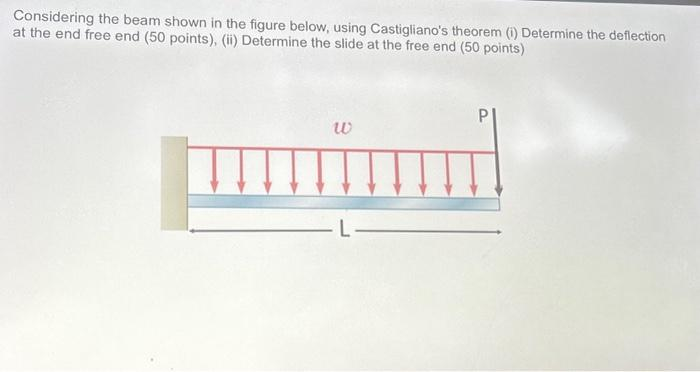Home / Expert Answers / Civil Engineering / considering-the-beam-shown-in-the-figure-below-using-castigliano-39-s-theorem-i-determine-the-deflec-pa571

# (Solved): Considering the beam shown in the figure below, using Castigliano's theorem (i) Determine the deflec ...

Considering the beam shown in the figure below, using Castigliano's theorem (i) Determine the deflection at the end free end (50 points), (ii) Determine the slide at the free end (50 points) w PConsidering the beam shown in the figure below, using Castigliano's theorem (i) Determine the deflection at the end free end (50 points), (ii) Determine the slide at the free end (50 points)

We have an Answer from Expert